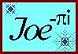Hip Shift and Hip Drop
Example Calculations

 The Hip Shift/Drop calculation determines the placement of the Hip rafter shoulders to permit the jack rafters and purlins to intercept or "plane" without backing the Hip.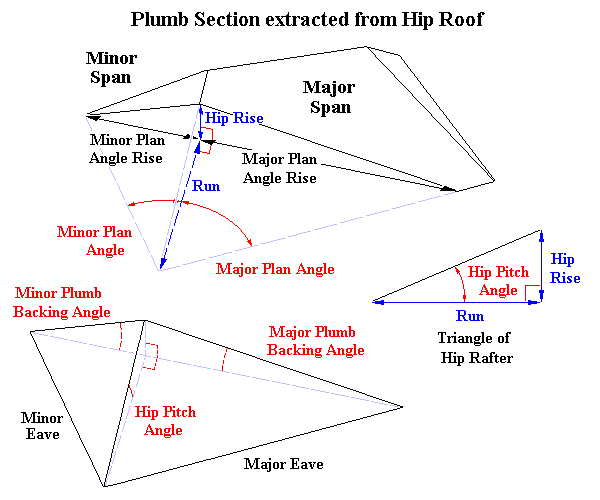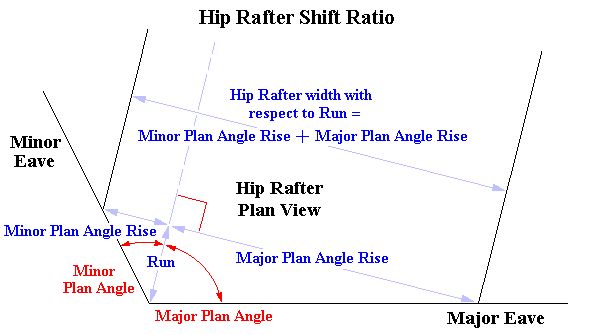Hip Shift Ratios in terms of Rise/Run The length labelled Run is equal for both Plan Angles Width of Hip Rafter to the Major Span side of the Hip Rafter = Major Plan Angle Rise Width of Hip Rafter to the Minor Span side of the Hip Rafter = Minor Plan Angle Rise Overall Width of Hip Rafter = Major Plan Angle Rise + Minor Plan Angle Rise The proportion of the Hip Rafter to the Major Span side of the Hip Rafter = Major Plan Angle Rise ÷ (Major Plan Angle Rise + Minor Plan Angle Rise) The proportion of the Hip Rafter to the Minor Span side of the Hip Rafter = Minor Plan Angle Rise ÷ (Major Plan Angle Rise + Minor Plan Angle Rise)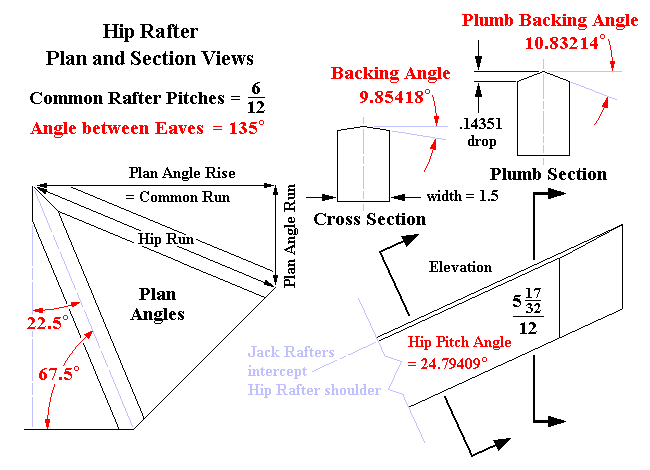The Hip rafters are symmetrical about their centerlines and need not be shifted. The Plumb Backing Angle may be determined using the following formulas: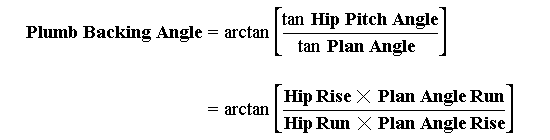Plumb Backing Angle Geometry and Formula
 Solving the Hip Drop Plumb Backing Angle = arctan (tan 24.79409° ÷ tan 67.50000°) = 10.83214° Hip Rafter Width = 1.5" Hip Drop = ½ Hip Rafter Width × tan Plumb Backing Angle Hip Drop = .75" × tan 10.83214° = .14351" ( approx. 5/32" ) Since the terms of the second formula within the parentheses equal tan Plumb Backing Angle the value of the angle need not be solved to find the Hip Drop. Plan Angle Rise = Common Run = 12 Plan Angle Run = 4.97056 Hip Rise = Common Rise = 6 Hip Run = 12.98871 Hip Drop = ½ Hip Rafter Width × tan Plumb Backing Angle Hip Drop = .75" × [(6 × 4.97056) ÷ (12.98871 × 12)] = .14351" ( approx. 5/32" )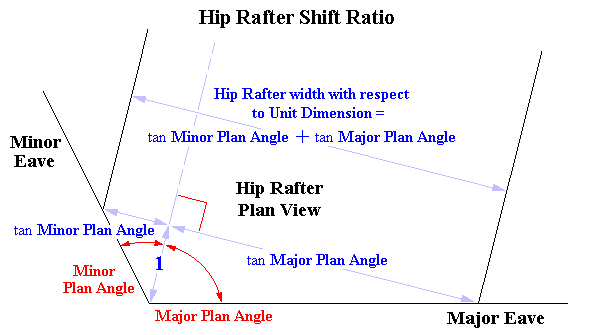Trigonometric Solution of Hip Shift Ratios A Unit Dimension is assigned to the Run Width of Hip Rafter to the Major Span side of the Hip Rafter = tan Major Plan Angle Width of Hip Rafter to the Minor Span side of the Hip Rafter = tan Minor Plan Angle Overall Width of Hip Rafter = tan Major Plan Angle + tan Minor Plan Angle The proportion of the Hip Rafter to the Major Span side of the Hip Rafter = tan Major Plan Angle ÷ (tan Major Plan Angle + tan Minor Plan Angle) The proportion of the Hip Rafter to the Minor Span side of the Hip Rafter = tan Minor Plan Angle ÷ (tan Major Plan Angle + tan Minor Plan Angle)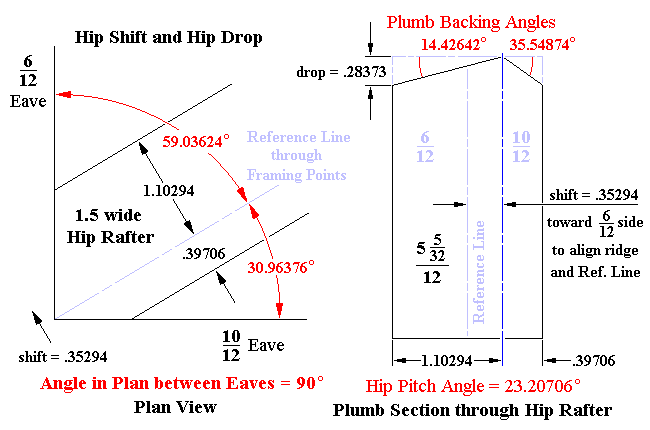Determining the Hip Shift/Drop Ratios 6/12 Plan Angle = 59.03624° 10/12 Plan Angle = 30.96376° 6/12 Shift Ratio = tan 59.03624° ÷ (tan 59.03624° + tan 30.96376° ) = .73529 10/12 Shift Ratio = tan 30.96376° ÷ (tan 59.03624° + tan 30.96376° ) = .26471 Hip Shift Ratio = (.73529 – .26471) ÷ 2 = .23529 or Hip Shift Ratio = .50000 – .26471 = .23529 or Hip Shift Ratio = .73529 – .50000 = .23529 The Hip rafter is shifted toward the 6/12 side of the roof 6/12 Pumb Backing Angle = 14.42642° 10/12 Pumb Backing Angle = 35.54874° Hip Drop Ratio = .26471 × tan 35.54874° = .18915 or Hip Drop Ratio = .73529 × tan 14.42642° = .18915 Adjusting the Ratios to the Hip Rafter Width Hip Rafter Width = 1.5" 6/12 Side Dimension = 1.5" × .73529 = 1.10294" ( approx. 1 3/32" ) 10/12 Side Dimension = 1.5" × .26471 = .39707" ( approx. 13/32" ) Hip Shift = 1.5" × .23529 = .35294" ( approx. 11/32" ) ... aligning the theoretical ridge line of the Hip rafter over the Hip run Hip Drop = 1.5" × .18915 = .28373" ( approx. 9/32" ) ... aligning the shoulders of the Hip rafter to permit the jack rafters/purlins to plane in at the same height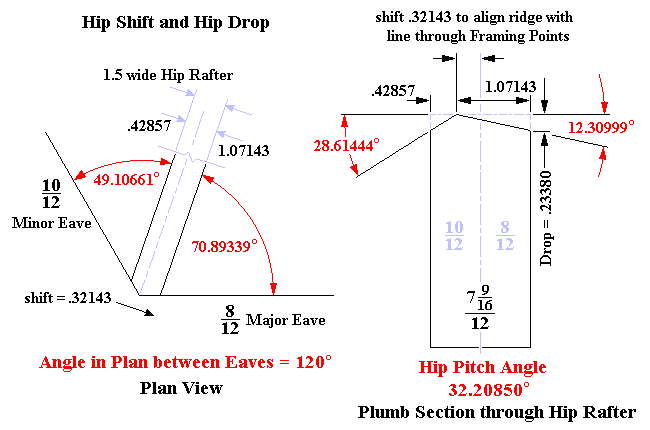Determining the Hip Shift/Drop Ratios 8/12 Plan Angle = 70.89339° 10/12 Plan Angle = 49.10661° 8/12 Shift Ratio = tan 70.89339° ÷ (tan 70.89339° + tan 49.10661° ) = .71429 10/12 Shift Ratio = tan 49.10661° ÷ (tan 70.89339° + tan 49.10661° ) = .28571 Hip Shift Ratio = (.71429 – .28571) ÷ 2 = .21429 or Hip Shift Ratio = .50000 – .28571 = .21429 or Hip Shift Ratio = .71429 – .50000 = .21429 The Hip rafter is shifted toward the 8/12 side of the roof 8/12 Pumb Backing Angle = 12.30999° 10/12 Pumb Backing Angle = 28.61444° Hip Drop Ratio = .28571 × tan 28.61444° = .15587 or Hip Drop Ratio = .71429 × tan 12.30999° = .15587 Adjusting the Ratios to the Hip Rafter Width Hip Rafter Width = 1.5" 8/12 Side Dimension = 1.5" × .71429 = 1.07143" ( approx. 1 1/16" ) 10/12 Side Dimension = 1.5" × .28571 = .42857" ( approx. 7/16" ) Hip Shift = 1.5" × .21429 = .32143" ( approx. 5/16" ) ... aligning the theoretical ridge line of the Hip rafter over the Hip run Hip Drop = 1.5" × .15587 = .23380" ( approx. 7/32" ) ... aligning the shoulders of the Hip rafter to permit the jack rafters/purlins to plane in at the same height